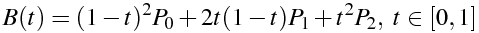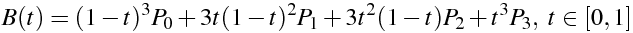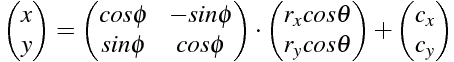Heya, Thanks for visiting!

# Curves and Arcs: Quadratic, Cubic, Elliptical (SVG implementations)

• canvas
• javascript
• c++
• svg

I've been playing around with Bezier curves and elliptical arcs for my on-going CNC plotter project. Instead of just using g-code commands which are standard on most CNC machines, I decided to support SVG path commands. This means supporting the linear moveTo(M, m) and lineTo(L, l) as well as curve(C, c, S, s -- Q, q, T, t)/arc(A, a) commands.

Here are a few articles to get you up to speed if you're unfamiliar with the SVG path commands:

## tl;dr, I just need a library

The JavaScript and C++ implementations are available on GitHub: SVG Curve Library.

I implemented the SVG path commands in JavaScript and ported it over to C++11 in order to run on the Teensy 3.0/3.1 (Arduino-like) microcontroller.

# Bezier Curves

Bezier curves are a made up of a set of control points, and a start/end point which are sometimes categorized along with the control points. The controls points influence where the curve should go. A Bbezier curve can have n number of control points but we will only go over the quadratic(1) and cubic(2) varieties. The math for Bezier curves is a simple parametric equation.

• A quadratic Bezier curve, has only a single control (1)point/handle.
• A cubic Bezier curve, has two control (2)points/handles.pointOnQuadraticBezierCurve: function(p0, p1, p2, t) {
function calculateQuadraticBezierParameter(x0, x1, x2, t) {
var result = Math.pow(1-t, 2)*x0 + 2*t*(1-t)*x1 + Math.pow(t, 2)*x2;

return result;
}

return {
};
},

## Cubic Bezier Math/CodepointOnCubicBezierCurve: function(p0, p1, p2, p3, t) {
function calculateCubicBezierParameter(x0, x1, x2, x3, t) {
var result = Math.pow(1-t, 3)*x0 + 3*t*Math.pow(1-t, 2)*x1 + 3*(1-t)*Math.pow(t, 2)*x2 + Math.pow(t, 3)*x3;

return result;
}

return {
x: calculateCubicBezierParameter(p0.x, p1.x, p2.x, p3.x, t),
y: calculateCubicBezierParameter(p0.y, p1.y, p2.y, p3.y, t)
};
},

A small collection of links that helped me.

# Elliptical Arcs

These were harder to implement for me, solely because of the amount of other information out there is very slim. I found the Implementation Notes page from the SVGWG to be most helpful.

Elliptical arcs are simply sub-sections(arcs) of an ellipse(oval).

## Elliptical Arc Math/Code

Elliptical arcs a bit more complicated to work out because the point on elliptical arc equation is not in a nice endpoint parameterization format. We will need to convert this center parameterized equation to a endpoint parameterization. If you want to do this conversion on your own, there are some great instructions from SVGWG.Beware: Matrix math, make sure to expand this expression correctly

• cx, cy are the coordinates of the center of the ellipse
• rx, ry are the radii of the ellipse (also known as its semi-major and semi-minor axes).
• phi (φ) is the rotation of the ellipse from the x-axis
• theta (θ) angle. The "actual" variable in the equation that determines where the point on the arc is.

		// http://www.w3.org/TR/SVG/implnote.html#ArcSyntax
// To double check our implementation against another source, you can use:
//      https://java.net/projects/svgsalamander/sources/svn/content/trunk/svg-core/src/main/java/com/kitfox/svg/pathcmd/Arc.java
//      http://fridrich.blogspot.com/2011/06/bounding-box-of-svg-elliptical-arc.html
// t: [0, 1]
pointOnEllipticalArc: function(p0, rx, ry, xAxisRotation, largeArcFlag, sweepFlag, p1, t) {

// In accordance to: http://www.w3.org/TR/SVG/implnote.html#ArcOutOfRangeParameters
rx = Math.abs(rx);
ry = Math.abs(ry);
xAxisRotation = mod(xAxisRotation, 360);
// If the endpoints are identical, then this is equivalent to omitting the elliptical arc segment entirely.
if(p0.x === p1.x && p0.y === p1.y) {
return p0;
}

// If rx = 0 or ry = 0 then this arc is treated as a straight line segment joining the endpoints.
if(rx === 0 || ry === 0) {
return this.pointOnLine(p0, p1, t);
}

// Following "Conversion from endpoint to center parameterization"
// http://www.w3.org/TR/SVG/implnote.html#ArcConversionEndpointToCenter

// Step #1: Compute transformedPoint
var dx = (p0.x-p1.x)/2;
var dy = (p0.y-p1.y)/2;
var transformedPoint = {
};
// Ensure radii are large enough
var radiiCheck = Math.pow(transformedPoint.x, 2)/Math.pow(rx, 2) + Math.pow(transformedPoint.y, 2)/Math.pow(ry, 2);
}

// Step #2: Compute transformedCenter
var cSquareNumerator = Math.pow(rx, 2)*Math.pow(ry, 2) - Math.pow(rx, 2)*Math.pow(transformedPoint.y, 2) - Math.pow(ry, 2)*Math.pow(transformedPoint.x, 2);
var cSquareRootDenom = Math.pow(rx, 2)*Math.pow(transformedPoint.y, 2) + Math.pow(ry, 2)*Math.pow(transformedPoint.x, 2);
// Make sure this never drops below zero because of precision
var cCoef = (largeArcFlag !== sweepFlag ? 1 : -1) * Math.sqrt(cRadicand);
var transformedCenter = {
x: cCoef*((rx*transformedPoint.y)/ry),
y: cCoef*(-(ry*transformedPoint.x)/rx)
};

// Step #3: Compute center
var center = {
};

// Step #4: Compute start/sweep angles
// Start angle of the elliptical arc prior to the stretch and rotate operations.
// Difference between the start and end angles
var startVector = {
x: (transformedPoint.x-transformedCenter.x)/rx,
y: (transformedPoint.y-transformedCenter.y)/ry
};
var startAngle = angleBetween({
x: 1,
y: 0
}, startVector);

var endVector = {
x: (-transformedPoint.x-transformedCenter.x)/rx,
y: (-transformedPoint.y-transformedCenter.y)/ry
};
var sweepAngle = angleBetween(startVector, endVector);

if(!sweepFlag && sweepAngle > 0) {
sweepAngle -= 2*Math.PI;
}
else if(sweepFlag && sweepAngle < 0) {
sweepAngle += 2*Math.PI;
}
// We use % instead of mod(..) because we want it to be -360deg to 360deg(but actually in radians)
sweepAngle %= 2*Math.PI;

// From http://www.w3.org/TR/SVG/implnote.html#ArcParameterizationAlternatives
var angle = startAngle+(sweepAngle*t);
var ellipseComponentX = rx*Math.cos(angle);
var ellipseComponentY = ry*Math.sin(angle);

var point = {
};

// Attach some extra info to use
point.ellipticalArcStartAngle = startAngle;
point.ellipticalArcEndAngle = startAngle+sweepAngle;
point.ellipticalArcAngle = angle;

point.ellipticalArcCenter = center;
point.resultantRx = rx;
point.resultantRy = ry;

return point;
},

A small collection of links that helped me.

# Arc/Curve Length (Distance)

Calculate the arc length/total distance of a curve/arc. It also returns some other useful/debug information that we use in generateLinearCurve(...)(see below).

Usage:

// Resolution is the number of segments we use to approximate
var resolution = 25;

var quadBezierArcLengthResult = approximateArcLengthOfCurve(resolution, function (t) {
});

var ellipticalArcArcLengthResult = approximateArcLengthOfCurve(resolution, function (t) {
return pointOnEllipticalArc(
startPoint,
rx,
ry,
xAxisRotation,
largeArcFlag,
sweepFlag,
endPoint,
t,
);
});

console.log('Elliptical arc, arc length:', ellipticalArcArcLengthResult.arcLength);

		approximateArcLengthOfCurve: function(resolution, pointOnCurveFunc) {
// Resolution is the number of segments we use
resolution = resolution ? resolution : 500;

var resultantArcLength = 0;
var arcLengthMap = [];
var approximationLines = [];

var prevPoint = pointOnCurveFunc(0);
var nextPoint;
for(var i = 0; i < resolution; i++) {
var t = clamp(i*(1/resolution), 0, 1);
nextPoint = pointOnCurveFunc(t);
resultantArcLength += distance(prevPoint, nextPoint);
approximationLines.push([prevPoint, nextPoint]);

arcLengthMap.push({
t: t,
arcLength: resultantArcLength
});

prevPoint = nextPoint;
}
// Last stretch to the endpoint
nextPoint = pointOnCurveFunc(1);
approximationLines.push([prevPoint, nextPoint]);
resultantArcLength += distance(prevPoint, nextPoint);
arcLengthMap.push({
t: 1,
arcLength: resultantArcLength
});

return {
arcLength: resultantArcLength,
arcLengthMap: arcLengthMap,
approximationLines: approximationLines
};
},

# Linear Curve/Arc

A challenge I faced, was dealing with t not being linear or having an even-distribution. This means that if you just sample t at a constant interval, you will have more samples bunched up in the curvier parts.

This at first seems good because you need the most detail in corners in order to accurately represent the curve, but it also leaves you with an unknown precision/error. So I decided to implement a linear curve function in order to get a known degree of accuracy. I am not sure if this is actually better or worse for accuracy/precision. The linear curve function is also useful in games or animations where you want the object to travel along a curve at a constant rate. This will work for any pointOnThing function.

generateLinearCurve(...) will return a new function where you can pass in a t [0, 1] and get a point on the curve back. Since t will be linear with this, 0.5 is halfway along the curve, distance wise.

Usage:

// Resolution is the number of segments we use to approximate
var resolution = 25;

var linearQuadBezier = generateLinearCurve(resolution, function (t) {
});

var linearEllipticalArc = generateLinearCurve(resolution, function (t) {
return pointOnEllipticalArc(
startPoint,
rx,
ry,
xAxisRotation,
largeArcFlag,
sweepFlag,
endPoint,
t,
);
});

// Pass in t [0, 1]
// Returns a point along the curve
var pt2 = linearEllipticalArc(0.5);

		// Returns a function that will linearly interpolate along the curve u: [0, 1]
// Inspired by: http://gamedev.stackexchange.com/a/5427/16587
generateLinearCurve: function(resolution, pointOnCurveFunc) {
// Resolution is the number of segments we use to approximate
resolution = resolution ? resolution : 500;

var result = this.approximateArcLengthOfCurve(resolution, pointOnCurveFunc);
var arcLength = result.arcLength;
var arcLengthMap = result.arcLengthMap;

// Transforms u[0-1] into a corresponding point along the curve. u * arcLength
var transformer = function(u) {
u = clamp(u, 0, 1);
var targetDistanceFromStartingPoint = u * arcLength;

var resultantT = 0;

var prevArcLength = 0;
var prevT = 0;
arcLengthMap.every(function(entry) {
var t = entry.t;
var arcLength = entry.arcLength;

// Once we go a past our target
// Lets interpolate from a previous to current
if(arcLength >= targetDistanceFromStartingPoint) {
var endDiff = arcLength - targetDistanceFromStartingPoint;
var startDiff = targetDistanceFromStartingPoint - prevArcLength;
var linearFactor = (startDiff/(endDiff+startDiff)) || 0;

resultantT = prevT + (t-prevT)*linearFactor;

// Break
return false;
}

prevArcLength = arcLength;
prevT = t;

return true;
});

return pointOnCurveFunc(resultantT);
};

transformer.arcLength = arcLength;

return transformer;
}
};